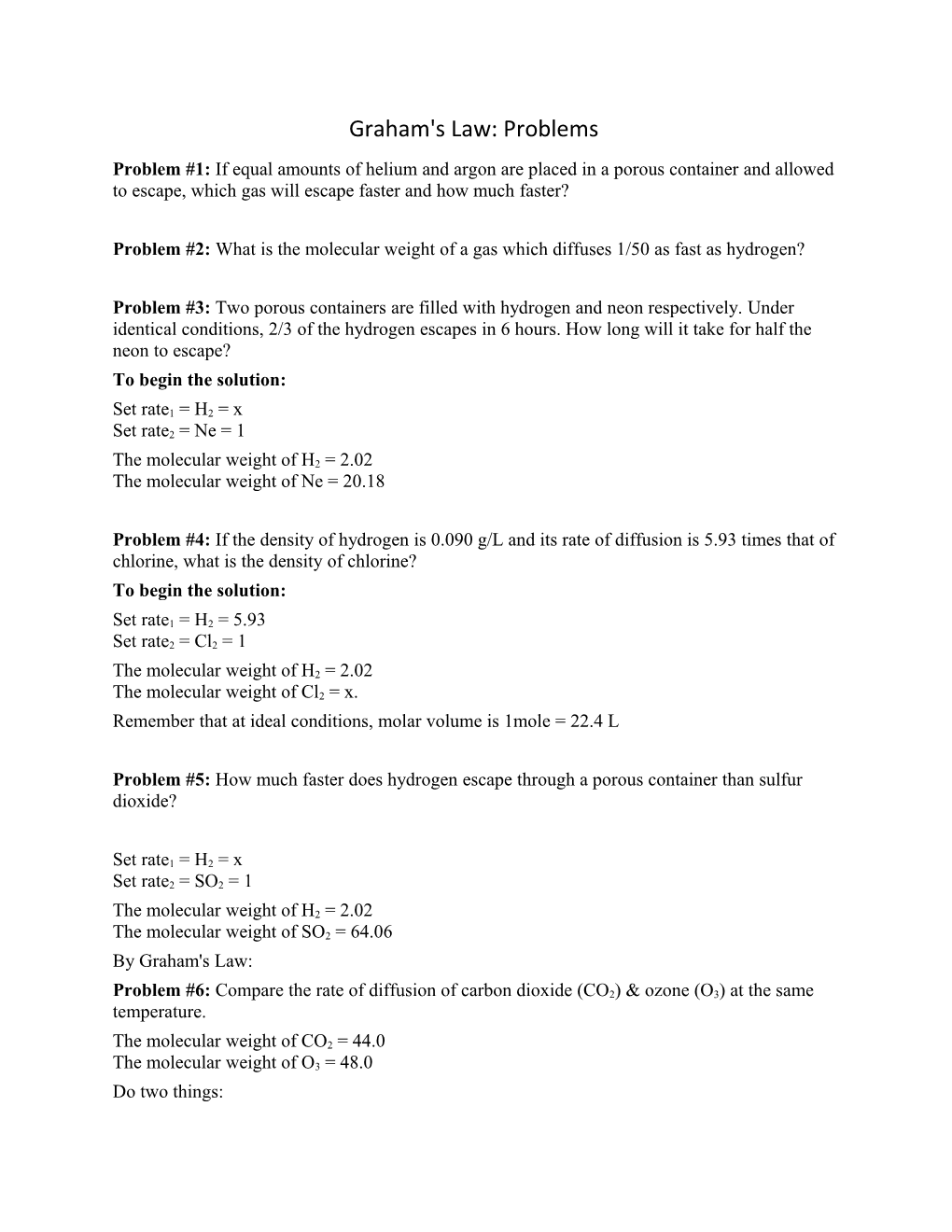# Problem #2: What Is the Molecular Weight of a Gas Which Diffuses 1/50 As Fast As Hydrogen?Graham's Law: Problems

Problem #1: If equal amounts of helium and argon are placed in a porous container and allowed to escape, which gas will escape faster and how much faster?

Problem #2: What is the molecular weight of a gas which diffuses 1/50 as fast as hydrogen?

Problem #3: Two porous containers are filled with hydrogen and neon respectively. Under identical conditions, 2/3 of the hydrogen escapes in 6 hours. How long will it take for half the neon to escape?

To begin the solution:

Set rate1 = H2 = x
Set rate2 = Ne = 1

The molecular weight of H2 = 2.02
The molecular weight of Ne = 20.18

Problem #4: If the density of hydrogen is 0.090 g/L and its rate of diffusion is 5.93 times that of chlorine, what is the density of chlorine?

To begin the solution:

Set rate1 = H2 = 5.93
Set rate2 = Cl2 = 1

The molecular weight of H2 = 2.02
The molecular weight of Cl2 = x.

Remember that at ideal conditions, molar volume is 1mole = 22.4 L

Problem #5: How much faster does hydrogen escape through a porous container than sulfur dioxide?

Set rate1 = H2 = x
Set rate2 = SO2 = 1

The molecular weight of H2 = 2.02
The molecular weight of SO2 = 64.06

By Graham's Law:

Problem #6: Compare the rate of diffusion of carbon dioxide (CO2) & ozone (O3) at the same temperature.

The molecular weight of CO2 = 44.0
The molecular weight of O3 = 48.0

Do two things:

set O2 rate = 1 (since it is the heavier)
assign it to be r2 (since r2 is in the denominator)

Problem #7: 2.278 x 10¯4mol of an unidentified gaseous substance effuses through a tiny hole in 95.70 s. Under identical conditions, 1.738 x 10¯4mol of argon gas takes 81.60 s to effuse. What is the molar mass of the unidentified substance?

Solution:

The first thing we need to do is compute the rate of effusion for each gas:

unknown gas: 2.278 x 10¯4mol / 95.70 s = 2.380 x 10¯6mol/s
argon: 1.738 x 10¯4mol / 81.60 s = 2.123 x 10¯6mol/s

Now, we are ready to use Graham's Law. Please note: (1) I will drop the 10¯6 from each rate and (2) we know the molar mass of argon from reference sources. Let argon be r1:

Problem #8: A compound composed of carbon, hydrogen, and chlorine diffuses through a pinhole 0.411 times as fast as neon. Select the correct molecular formula for the compound:

a) CHCl3
b) CH2Cl2
c) C2H2Cl2
d) C2H3Cl

Solution:

Let r1 = 0.411; this means r2 (the rate of effusion for Ne) equals 1.

Inserting values into Graham's Law yields:

0/411 / 1 = √(20.18 /x )

the 20.18 is the atomic weight of Ne.

Squaring both sides gives:

0.16892 = 20.18 / x

Solving for x yields:

x = 119.46 g/mol

Examining the formulas for the possible answers, What chemical gives a molecular weight of about 119.5.

Problem #9: If a molecule of CH4 diffuses a distance of 0.530 m from a point source, calculate the distance (in meters) that a molecule of N2 would diffuse under the same conditions for the same period of time.

Solution:

Assume the gases each diffuse in one second, in order to create a rate.

Set rate1 = N2 = x
Set rate2 = CH4 = 0.530 m/s

The molecular weight of N2 = 28.0
The molecular weight of CH4 = 16.0